帐号 自动登录 找回密码 密码 立即注册→加入我们
 搜索 热搜: 下载 VB C 实现 编写

【搬运】如何理解stdlib.h里的_countof()宏17主题 529积分

UID
140

5

30 点

376 个

26 次

0 份

44 小时

2014-3-22发表于 2015-7-19 20:15:49 | 显示全部楼层 |阅读模式

欢迎访问技术宅的结界，请注册或者登录吧。

x

[C++] 纯文本查看 复制代码
extern "C++"
{
template <typename _CountofType, size_t _SizeOfArray>
char (*__countof_helper(UNALIGNED _CountofType (&_Array)[_SizeOfArray]))[_SizeOfArray];

#define _countof(_Array) sizeof(*__countof_helper(_Array))
}

[C] 纯文本查看 复制代码
#include <stdlib.h>

int main()
{
int a;
......
int arraySize = _countof(a); //得到数组a的大小
}
////////////////////////////////////////////////////////////////////////////////////////////////

[C++] 纯文本查看 复制代码
template <typename T, size_t N>
char (* __countof_helper ( T (&_Array)[N] )  )[N];

__countof_helper是一个函数（红色部分）；

1) 数组引用：

T (&)[N] (注意：有个括号)。比如：
int a = {0};
int (&ra) = a; 这里ra就是一个对数组a的引用，其这个数组的大小也是5；

int a = {0};
int (&ra) = a; //编译错误：error C2440: 'initializing' : cannot convert from 'int ' to 'int (&)'

2) 数组指针：

T (*)[N] (注意：有个括号)。比如：
[C] 纯文本查看 复制代码
int a;
int (*p) = &a;// 是一个指针，该指针指向“一个有5个元素的数组”；简称为“数组指针”

[C] 纯文本查看 复制代码
int a;
int (*p) = &a;//error C2440: '=' : cannot convert from 'int (*)' to 'int (*)'

[C] 纯文本查看 复制代码
char * names[]=
{
"Mike",
"John",
"Tom"
};
names就是一个指针数组，该数组有3个元素，每个元素都是一个char类型指针，指向一个字符串。

[C] 纯文本查看 复制代码
int a; int b;
int * p = 0;
p = a; // ok
p = b; // ok
3) 函数返回“数组指针”的声明语法：

T (* Fun( param_list ) )[N];
Fun是一个函数，其返回值类型是 T (*)[N];

T (*)[N] Fun( param_list ); 这么声明多好理解啊。但是这么是不行的。
C++语法比较复杂，语言设计者对(),[],*,&,&&这些符号所放的位置有特殊的要求。
<<Thinking in C++>>的第3章有一节“Aside: complicated declarations & definitions”，举了一些复杂声明语法的例子。

sizeof是C/C++语言中的keyword，不是函数。对其参数里的表达式是不会在执行期去执行的，而只是在编译期去推算整个表达式的最后的类型信息。

[C] 纯文本查看 复制代码
int Fun(); // only declare it without definition
sizeof( Fun() );  // 这里Fun()函数不会在运行的时候被执行，仅仅是在编译时，编译器需要知道的是：Fun()函数返回值的类型是什么。

[C++] 纯文本查看 复制代码
template<int x, int y>
struct sum_
{
enum { value = x + y };
};
int sum = sum_<3, 4>::value; // sum的值在编译期间就已经确定下来了，不是在执行期间由CPU运算得到。

[C++] 纯文本查看 复制代码
template< typename T, size_t N>
void Fun( T (&)[N] );

[C] 纯文本查看 复制代码
int a;
Fun(a); // 编译器经过推导就知道，T=int，N=5。注意这里的N能得到5，是因为Fun的参数声明决定的。这里Fun的参数是：数组引用。

[C] 纯文本查看 复制代码
int * p = NULL;
Fun( p ); // error C2784: 'void Fun(T (&)[N])' : could not deduce template argument for 'T (&)[N]' from 'int *'

【题外话：模板是泛型编程的基础。利用模板自动推导的能力，可以构建出很有技巧性的代码实现。比如：Boost库。更甚者：Loki库<Modern C++ Design>(中文名：C++设计新思维)这本书附带的库。关于模板的语法和自动推导，可以看<C++ Templates the complete guide>，大陆翻译的中译本编译的不好。侯捷翻译的《C++ Templates全览》比较好，网上有电子的pdf可以找到，但是只能在电脑上看，不能打印，也不能copy里面的文字。】

[C++] 纯文本查看 复制代码
template <typename _CountofType, size_t _SizeOfArray>
char (*__countof_helper(UNALIGNED _CountofType (&_Array)[_SizeOfArray]))[_SizeOfArray];
#define _countof(_Array) sizeof(*__countof_helper(_Array))
a) #define里的sizeof()的参数是 “*__countof_helper(_Array)”（注意：有个*），而不是“__countof_helper(_Array)”，

sizeof(一个指针)和sizeof(一个数组)，是有很大的区别的。看下面的例子：
[C] 纯文本查看 复制代码
int a;
int *p = a;
int (*pa) = &a;

// p和pa都是指针，但是指针的类型不同：p的类型是int*；pa的类型是int(*)。

sizeof(p)       // = 4;
sizeof(a)       // = 20 = 5*sizeof(int)
sizeof(*p)     // = sizeof(int) = 4;
sizeof(pa)    // = 4;
sizeof(*pa)  // = sizeof(a) = 20;
C/C++对于数组和指针是明确区分其类型的。数组的信息包括(类型+大小)，而指针则没有大小信息。

[C] 纯文本查看 复制代码
void Fun( int a )
{
int n = sizeof(a);  // n为4，相对于就是sizeof(int*).因为编译器只传递过来了数组的地址，大小信息没有了。
}

[C] 纯文本查看 复制代码
void Fun( int a );
void Fun( int a[] );
void Fun( int *a );

void Fun( int a[], int size );

b)__countof_helper函数的类型是char，而不是_CountofType。因为sizeof( * (char(*)[N]) )【注：这么写只是为了示范说明，在代码里不能这么写，通不过编译的】 刚好等于N，因为sizeof(char)在任何操作系统上都是=1；

c) 这个模板函数只有声明(declaration)，没有定义(definition)。没有定义体的原因是：sizeof是在编译期完成的，不需要具体的定义体出现（上面第二点里说了）。同时，模板推导也是在编译期完成的。所以_countof()整个宏在编译期就完成了。不需要函数的具体实现体。如果定义了实现体，_countof依然能工作，但是那些实现体都是不会被用到的，而这些实现体会在程序运行起来之后，加载到内存里。这样就浪费内存了。

[C] 纯文本查看 复制代码
int a;
__countof_helper(a); // 能编译通过，但是链接时，链接器会告诉你找不到实现体。
[C++] 纯文本查看 复制代码
template <typename T, size_t N>
char (&ArraySizeHelper(T (&array)[N]))[N];
#define arraysize(array) (sizeof(ArraySizeHelper(array)))

1) ArraySizeHelper函数返回的是 char (&)[N]，而不是char (*)[N]；
2) #define里没有*号。因为sizeof( char (&)[N] ) = N;

“We could return a reference instead of a pointer but older compilers do not accept that.”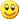That's it.

winnt.h 里的注释说明的很好，值得看看。摘抄如下：
[C++] 纯文本查看 复制代码
//
// RtlpNumberOf is a function that takes a reference to an array of N Ts.
//
// typedef T array_of_T[N];
// typedef array_of_T &reference_to_array_of_T;
//
// RtlpNumberOf returns a pointer to an array of N chars.
// We could return a reference instead of a pointer but older compilers do not accept that.
//
// typedef char array_of_char[N];
// typedef array_of_char *pointer_to_array_of_char;
//
// sizeof(array_of_char) == N
// sizeof(*pointer_to_array_of_char) == N
//
// pointer_to_array_of_char RtlpNumberOf(reference_to_array_of_T);
//
// We never even call RtlpNumberOf, we just take the size of dereferencing its return type.
// We do not even implement RtlpNumberOf, we just decare it.
//
// Attempts to pass pointers instead of arrays to this macro result in compile time errors.
// That is the point.
//
extern "C++" // templates cannot be declared to have 'C' linkage
template <typename T, size_t N>
char (*RtlpNumberOf( UNALIGNED T (&)[N] ))[N];

#define RTL_NUMBER_OF_V2(A) (sizeof(*RtlpNumberOf(A)))
P.S. 下面这个链接值得看。其中的解释相当好。
http://blogs.msdn.com/the1/archive/2004/05/07/128242.aspx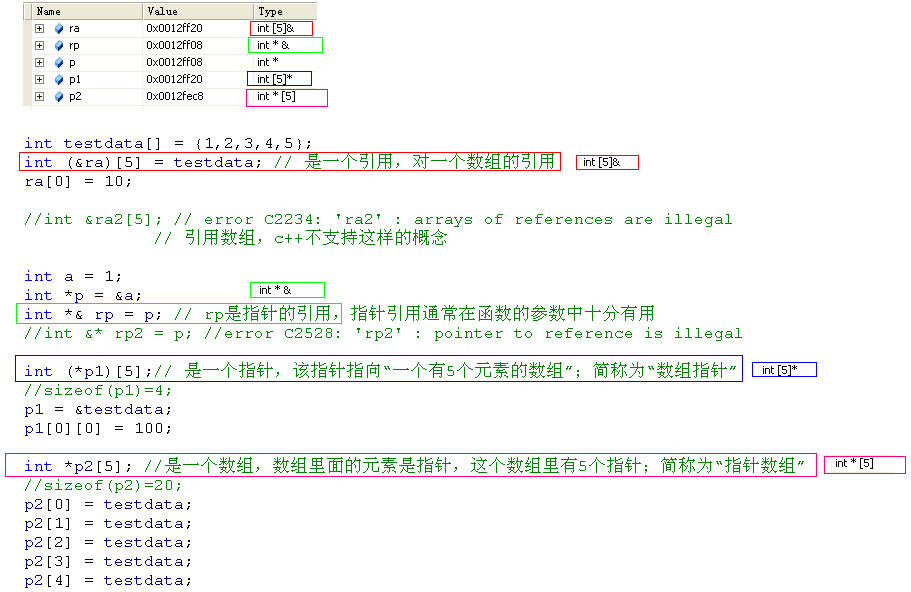1044主题 5万积分

UID
1

217

294 点

18288 个

37113 次

0 份

1742 小时

2014-1-26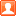发表于 2015-7-19 21:35:08 | 显示全部楼层
 ……这是在C里面使用C艹

 本版积分规则 回帖并转播 回帖后跳转到最后一页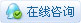|申请友链||Archiver|手机版|小黑屋|技术宅的结界 ( 滇ICP备16008837号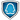|网站地图

GMT+8, 2019-10-14 11:19 , Processed in 0.118271 second(s), 39 queries , Gzip On.